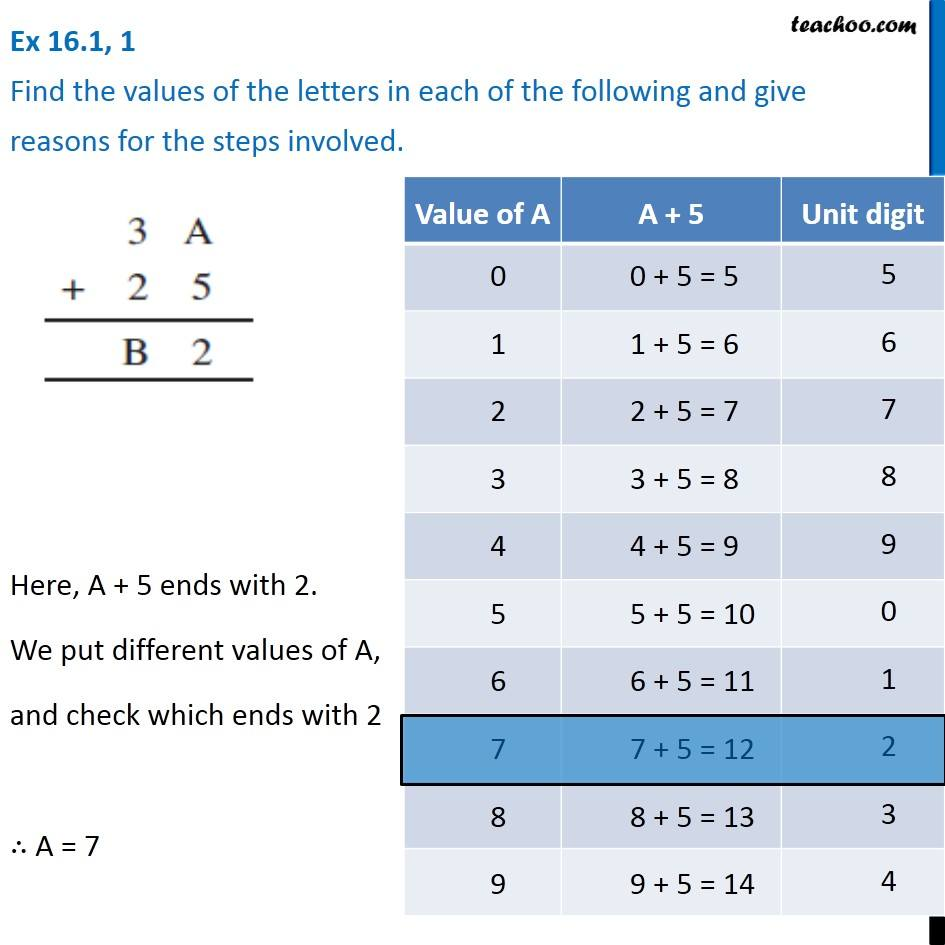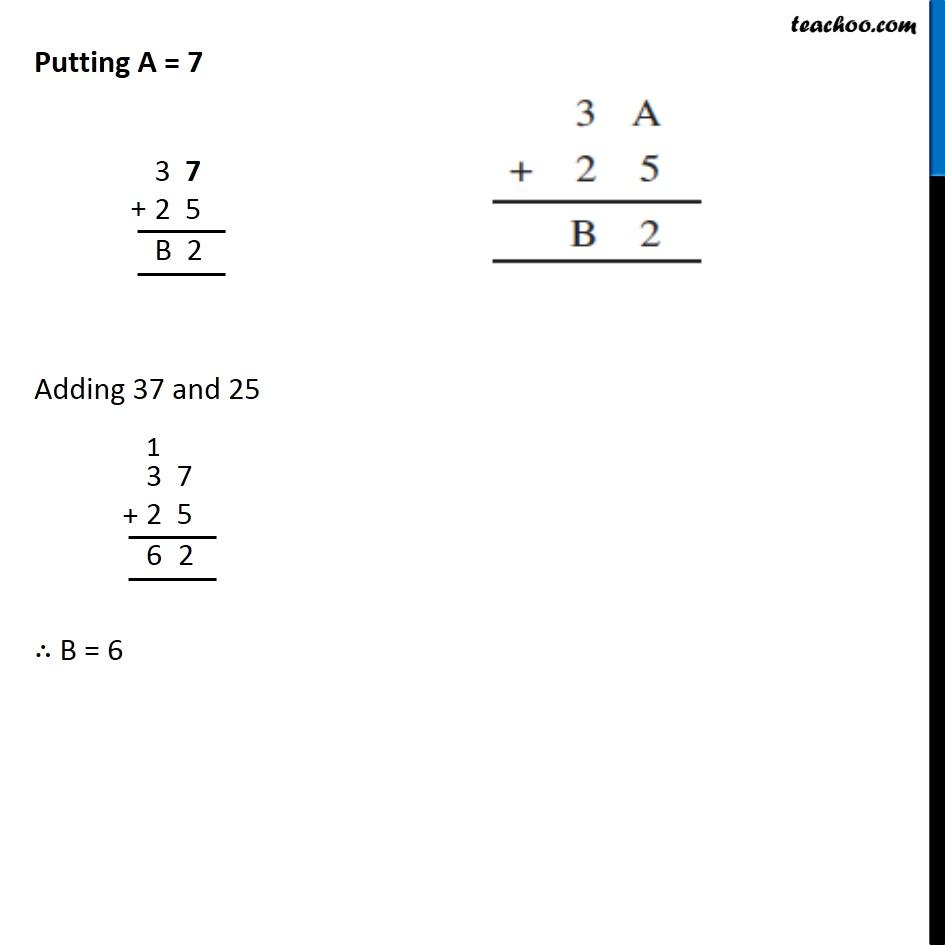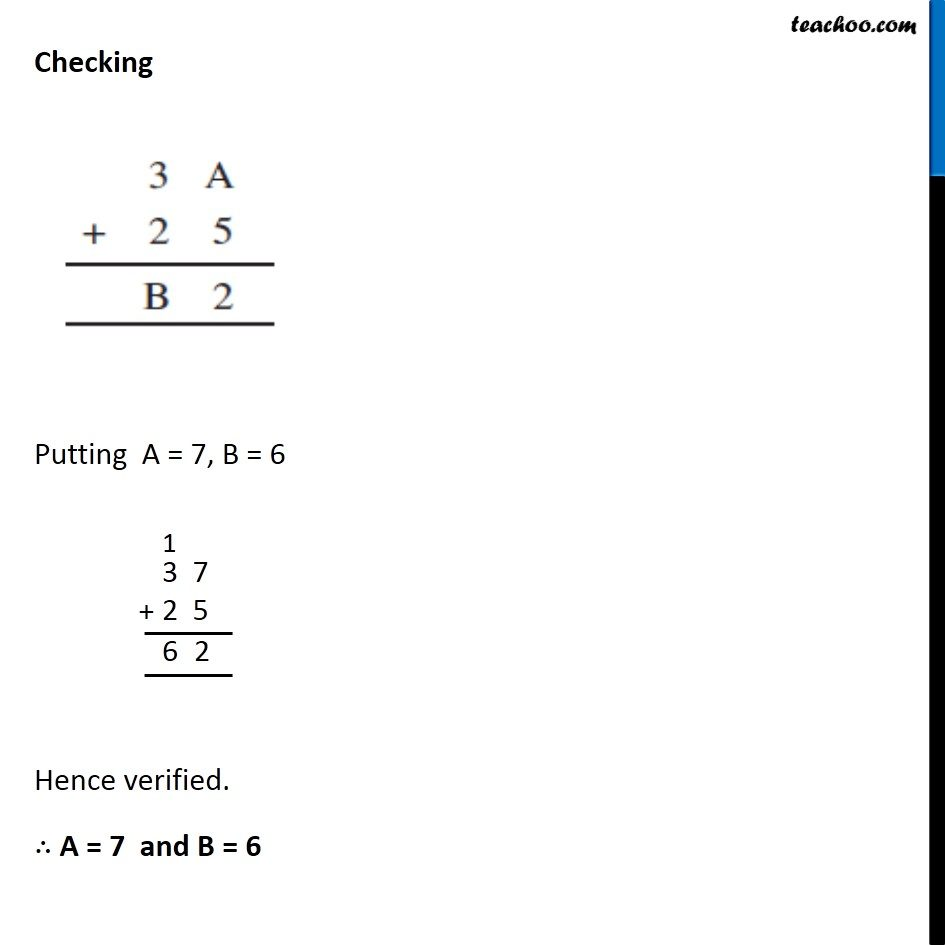Letters for Digits - Addition Questions

Playing with Numbers
Concept wiseLearn in your speed, with individual attention - Teachoo Maths 1-on-1 Class

### Transcript

Question 1 Find the values of the letters in each of the following and give reasons for the steps involved. Here, A + 5 ends with 2. We put different values of A, and check which ends with 2 ∴ A = 7 Putting A = 7 Adding 37 and 25 ∴ B = 6 Putting A = 7 Adding 37 and 25 ∴ B = 6 Checking Putting A = 7, B = 6 Hence verified. ∴ A = 7 and B = 6